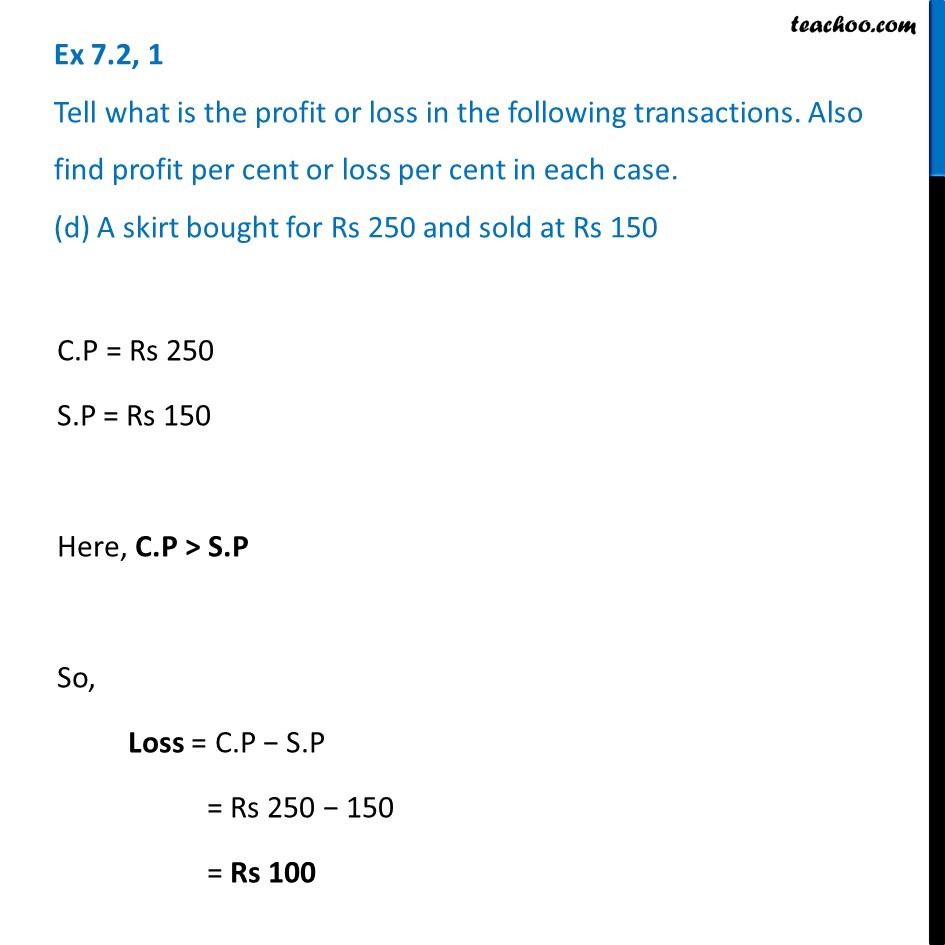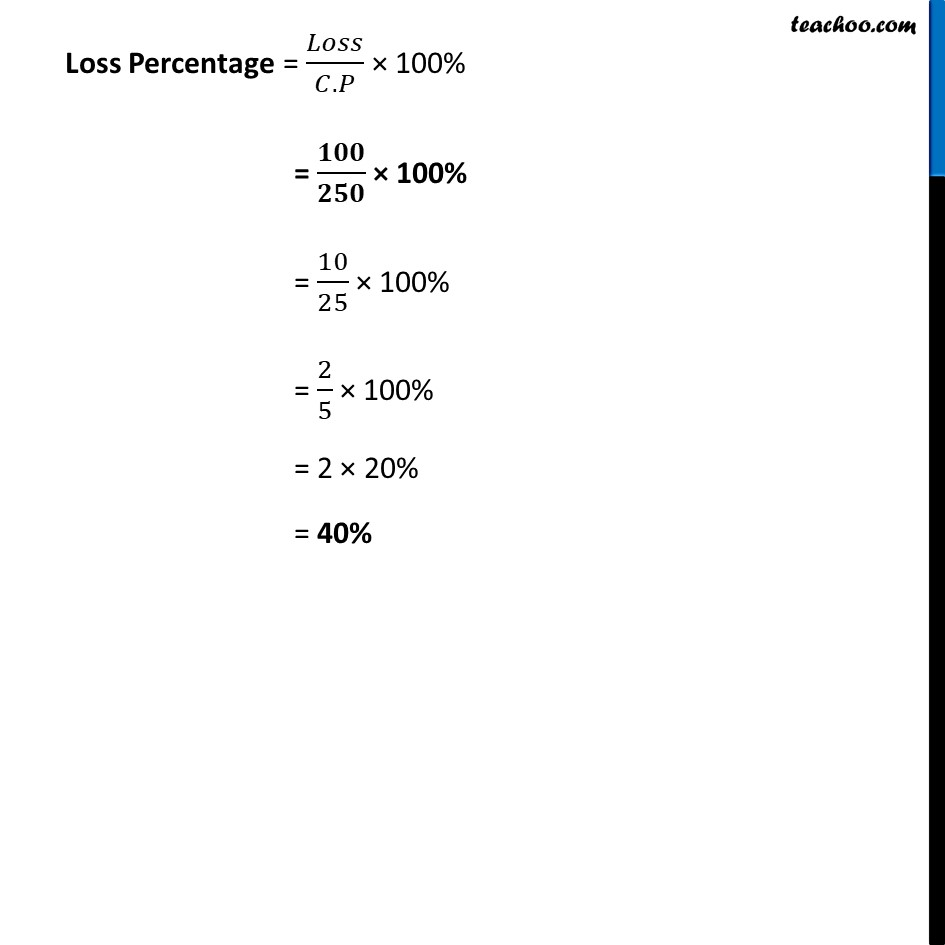Ex 7.2

Chapter 7 Class 7 Comparing Quantities
Serial order wiseLearn in your speed, with individual attention - Teachoo Maths 1-on-1 Class

### Transcript

Ex 7.2, 1 Tell what is the profit or loss in the following transactions. Also find profit per cent or loss per cent in each case. (d) A skirt bought for Rs 250 and sold at Rs 150 C.P = Rs 250 S.P = Rs 150 Here, C.P > S.P So, Loss = C.P − S.P = Rs 250 − 150 = Rs 100 Loss Percentage = 𝐿𝑜𝑠𝑠/(𝐶.𝑃) × 100% = 𝟏𝟎𝟎/𝟐𝟓𝟎 × 100% = 10/25 × 100% = 2/5 × 100% = 2 × 20% = 40%